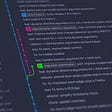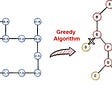# Faster Indexing in Tables, datetime Arrays, and Other Data Types

## By Loren Shure

Today I’d like to introduce a guest blogger, Stephen Doe, who works for the MATLAB Documentation team here at MathWorks. In today’s post, Stephen discusses how to take advantage of recent performance improvements when indexing into tables. The same approach applies to many different data types. While the release notes describe the performance improvements, in today’s post Stephen also offers further advice based on a simple code example.

# Contents

• So, What Has Improved, and How?
• Assign Table Elements in for-Loop
• Scripts and try-catch Considered Harmful
• Best Practices
• Code Samples

# So, What Has Improved, and How?

As of R2020a, the MATLAB data types team has delivered substantial performance improvements for indexing into certain types of arrays. The improved performance comes from in-place optimizations. They are most apparent when you access or assign values to many array elements within a for-loop. The improved data types are:

The improvements were delivered over two releases (R2019b and R2020a). So, in this post I compare the performance of R2020a to R2019a, the latest release without any of these improvements. Overall, we see that for these data types, assignments to array elements are many times faster than in R2019a.

• 1.5–2x faster when assigning to elements of a table or timetable variable
• Order of magnitude faster (at least) when assigning to elements of calendarDuration, categorical, datetime, and duration arrays

You can find the full details, including test code that illustrates the performance improvements between releases, in the release notes. As it happens, we have a new format for describing performance enhancements with more quantitative detail. These links take you directly to the performance release notes for R2019b and R2020a:

With that kind of detail, is there more that needs to be said? Yes. I’m going to show you how best to take advantage of these performance improvements. I’m also going to explain some circumstances where these improvements don’t take effect.

# Assign Table Elements in for-Loop

Here’s a simple example that takes full advantage of the indexing performance improvements. In this example, I calculate the position of a projectile at regular time steps. I use a formula where the position and velocity at each step depend on the position and velocity of the previous step. I create a table and I assign the positions and velocities to rows of the table using a for-loop. The figure shows the path taken by the projectile in this example.

Here is a function, named projectile, that calculate positions and velocities and then assigns them to a table. (It depends on another function, step, that calculates position and velocity at one time step.) Note that in the for-loop, I use dot notation to access the table variables. Then I use the loop counter to index into the variables (T.x(i), T.y(i), and so on). (I’ve attached the projectile and step functions to the end of this blog post.)

`function T = projectile(v,angle)% PROJECTILE.M - Function to create table of projectile positions and% velocities under free fall.dt = 0.001;    % 0.001 secondsT = table('Size',[30/dt 4],'VariableTypes',["double","double","double","double"],'VariableNames',["x","y","vx","vy"]);T{1,:} = [0 0 v*cosd(angle) v*sind(angle)];for i = 2:height(T)    [T.x(i), T.y(i), T.vx(i), T.vy(i)] = step(T.x(i-1), T.y(i-1), T.vx(i-1), T.vy(i-1), dt);endend`

Let’s call the projectile function with a starting velocity of 50 m/s and an angle of 45 degrees. With this call, the output table has 30,000 rows. I’ll use the head function to display the first five rows.

`T = projectile(50,45);head(T,5)ans =  5×4 table       x           y          vx        vy      ________    ________    ______    ______           0           0    35.355    35.355    0.035355    0.035341    35.355    35.346    0.070711    0.070671    35.355    35.336     0.10607     0.10599    35.355    35.326     0.14142      0.1413    35.355    35.316`

Now let’s use tic and toc to estimate the execution time for this function in two different versions of MATLAB: R2019a (before recent improvements) and R2020a.

`ticT = projectile(50,45);toc`

R2019a: 13.06 seconds

R2020a: 7.64 seconds.

(I made both calls on the same machine: a Windows 10, Intel® Xeon® W-2133 @ 3.60 GHz test system.)

This code is about 1.70x times faster in R2020a! And you’ll see performance improvements that are at least this good with very large tables and timetables (millions of rows), and also in categorical and datetime arrays.

Now, note that I wrote this example in such a way as to force use of a for-loop. If you can, the best strategy of all is to vectorize your code for the best performance. “Vectorizing” code pretty much means operating on arrays instead of elements of an array. For example, it’s much more efficient to call z = x + y instead of calling z(i) = x(i) + y(i) in a for-loop.

But if you have code that you can’t vectorize, because it accesses many other table or array elements — like the code in my example — then the performance improvements for these data types will help your code run faster.

So now we’re good to go, right? Not so fast.

# Scripts and try-catch Considered Harmful

All right, harmful is an exaggeration. It’s perfectly fine to put your code in a script, or within a try-catch block, or to work with variables in the workspace. In all these cases, the code I wrote for the projectile function returns the exact same table.

But if that code is in a script or a try-catch block, or if you are interactively working with workspace variables, then there is no performance enhancement. For example, let me rewrite the code as a script, in the file projectile_script.m. Within the script, I assign the same starting velocity and angle as above. (I’ve attached a copy of projectile_script.m to the end of this blog post.)

`% PROJECTILE_SCRIPT.M - Script that creates table of projectile positions% and velocities under free fall.dt = 0.001;    % 0.001 secondsv = 50;        % 50 m/sangle = 45;    % 45 degreesT = table('Size',[30/dt 4],'VariableTypes',["double","double","double","double"],'VariableNames',["x","y","vx","vy"]);T{1,:} = [0 0 v*cosd(angle) v*sind(angle)];for i = 2:height(T)    [T.x(i), T.y(i), T.vx(i), T.vy(i)] = step(T.x(i-1), T.y(i-1), T.vx(i-1), T.vy(i-1), dt);end`

Now I call the function and time it:

`ticprojectile_scripttoc`

R2019a: 12.36 seconds

R2020a: 11.83 seconds

The difference between the two releases is now much smaller! What happened?

Well, it’s complicated. In essence, MATLAB applies in-place optimizations to the indexing done in this line of code:

`[T.x(i), T.y(i), T.vx(i), T.vy(i)] = step(T.x(i-1), T.y(i-1), T.vx(i-1), T.vy(i-1), dt);`

To take advantage of the in-place optimizations for these data types, you must perform the indexing within a function. If you do so with workspace variables or within a script, you won’t see the full performance improvement.

Also, the improvement is lost when the indexing code is within a try-catch block, even when that block is itself within a function. But in such cases, you can get the performance back by putting the indexing code into a separate function.

I won’t go into the full details about try-catch blocks in this post. However, I have attached two files to the end of this post, projectile_try_catch.m and projectile_try_regained.m. These files show the problem and its workaround.

To sum up, this table shows the different pieces of code that I wrote for this post, and the performance you can expect in each case.

# Best Practices

To sum up, here are the best practices to keep in mind to get best performance when writing code for data types such as table, datetime, and categorical:

• DO vectorize code when you can. For example, operate on table variables (T.X) instead of elements of table variables (T.X(i))
• DO put your table, datetime, and categorical indexing code in functions, if you’re doing a lot of indexing and can’t vectorize your code.
• AVOID scripts, at least for code that does a lot of indexing. Put the indexing code in a function.
• AVOID try-catch blocks for code that does a lot of indexing. Put it in its own function.

Since the MATLAB data types team continues to work on improving performance, we’d love to hear more about your experience with our data types. Please tell us more about your challenges using tables, timetables, datetime, or categorical arrays here.

# Code Samples

`function [x, y, vx, vy] = step(x,y,vx,vy,dt)% STEP.M - Calculate position and velocity based on input position, % velocity, and local gravitational acceleration. Positions in meters,% velocities in m/s, dt in seconds. g = -9.8;  % -9.8 m/s^2vy = vy + g*dt;y = y + vy*dt + (g/2)*dt^2;x = x + vx*dt;endfunction T = projectile(v,angle)% PROJECTILE.M - Function to create table of projectile positions and% velocities under free fall.dt = 0.001;    % 0.001 secondsT = table('Size',[30/dt 4],'VariableTypes',["double","double","double","double"],'VariableNames',["x","y","vx","vy"]);T{1,:} = [0 0 v*cosd(angle) v*sind(angle)];for i = 2:height(T)    [T.x(i), T.y(i), T.vx(i), T.vy(i)] = step(T.x(i-1), T.y(i-1), T.vx(i-1), T.vy(i-1), dt);endend% PROJECTILE_SCRIPT.M - Script that creates table of projectile positions% and velocities under free fall.dt = 0.001;    % 0.001 secondsv = 50;        % 50 m/sangle = 45;    % 45 degreesT = table('Size',[30/dt 4],'VariableTypes',["double","double","double","double"],'VariableNames',["x","y","vx","vy"]);T{1,:} = [0 0 v*cosd(angle) v*sind(angle)];for i = 2:height(T)    [T.x(i), T.y(i), T.vx(i), T.vy(i)] = step(T.x(i-1), T.y(i-1), T.vx(i-1), T.vy(i-1), dt);endfunction T = projectile_try_catch(v,angle)% PROJECTILE_TRY_CATCH.M - A copy of PROJECTILE.M, but with a try-catch% block. R2020a in-place optimizations are LOST because of the try-catch% block.dt = 0.001;    % 0.001 secondsT = table('Size',[30/dt 4],'VariableTypes',["double","double","double","double"],'VariableNames',["x","y","vx","vy"]);try    T{1,:} = [0 0 v*cosd(angle) v*sind(angle)];    for i = 2:height(T)        [T.x(i), T.y(i), T.vx(i), T.vy(i)] = step(T.x(i-1), T.y(i-1), T.vx(i-1), T.vy(i-1), dt);    endcatchendendfunction T = projectile_try_regained(v,angle)% PROJECTILE_TRY_REGAINED.M - A copy of PROJECTILE.M, but with a try-catch% block placed in a separate function. R2020a in-place optimizations are% REGAINED because the try-catch block is in a separate, local function.try    T = projectile_local(v,angle);catchendendfunction T = projectile_local(v,angle)% This is the code that creates the table for PROJECTILE_TRY_REGAINED.% For best performance, do not use try-catch here, but rather in the% calling function.dt = 0.001;T = table('Size',[30/dt 4],'VariableTypes',["double","double","double","double"],'VariableNames',["x","y","vx","vy"]);    T{1,:} = [0 0 v*cosd(angle) v*sind(angle)];for i = 2:height(T)    [T.x(i), T.y(i), T.vx(i), T.vy(i)] = step(T.x(i-1), T.y(i-1), T.vx(i-1), T.vy(i-1), dt);endend`

Published with MATLAB® R2020a

Originally published at https://blogs.mathworks.com on April 30, 2020.

--

--

--

## More from MathWorks

We at MathWorks believe in the importance of engineers and scientists. They increase human knowledge and profoundly improve our standard of living. We created MATLAB and Simulink to help them do their best work.

## Top Feature of DataFrame often Under-Utilized, Markdown## Predicting the Next Baseball Pitch: Utilizing machine learning to gain an advantage## Why Aren’t We Talking About Experiment Management as Much as We Should Be?## Getting Started with Git and GitHub: A Complete Tutorial for Beginner## What Causes Fatal City Crashes?## Yogscast Jingle Jam Donation Analysis## Production ML: Getting Started with MLOps## Greedy Algorithm in Python## Using python poetry with .pypirc## Math: C# vs Python, Which is better?